# Preliminary evaluation of the expansion system size for a pressurized gas loop: application to a fusion reactor based on a helium-cooled blanket

Preliminary evaluation of the expansion system size for a pressurized gas loop: application to a fusion reactor based on a helium-cooled blanket

Gianfranco CarusoMatteo Nobili

Sapienza, University of Rome, Department of Astronautical, Electrical and Energy Engineering, Nuclear Section - C.so Vittorio Emanuele II, 244 – 00186 Rome, Italy

Corresponding Author Email:
gianfranco.caruso@uniroma1.it
Page:
211-218
|
DOI:
https://doi.org/10.18280/ijht.350128
|
Accepted:
|
Published:
31 March 2017
| Citation

OPEN ACCESS

Abstract:

Some considerations to preliminarily design the size of the Expansion Volume (EV) and the relief pipes for a Vacuum Vessel Pressure Suppression System, to be adopted in a fusion reactor based on a helium cooled blanket, are presented. The volume of the EV depends on the total energy of the cooling system and it can be sized based on a required final pressure at equilibrium, by a simple energy balance. Two different EV solutions have been analysed: a “dry” EV and a “wet” EV. In this last, a certain amount of water could be mixed (by spraying or discharging in a pool) with the discharged helium, to reduce its temperature and allowing a lower size of the EV with respect to the “dry” solution. The pressure peak in vacuum vessel (VV) depends mainly on break area and flow area of the relief pipes and a simple formula to be used to size these pipes is suggested. The computer code CONSEN has been used to perform sensitivity analyses and to verify the methodology.

Keywords:

Pressure Suppression System, Fusion Reactor, Helium, Safety Analysis, Expansion Volume

1. Introduction

In a fusion reactor, an important safety issue is the overpressure resulting from an in-vessel loss of coolant accident (LOCA). In water cooled systems, overpressure resulting from a loss of coolant (e.g. steam in the vacuum vessel) can be mitigated by re-condensing the steam in a pressure suppression pool. The inability to do so for helium cooled systems implies a need for a large expansion volume to accommodate a helium LOCA and initial studies point to the need of a very large expansion volume .

This can be partially mitigated by dividing the helium coolant system into separate loops: a design-basis pipe break accident, then, implies a partial loss of the coolant inventory. Still, since the vacuum vessel is not expected to be able to withstand high pressures, it must be equipped with a rupture disk in order to vent to an adequate expansion volume. This volume serves as a secondary confinement boundary, and it have to be designed to a given size and maximum pressure based on the coolant inventory it must accommodate. In past ARIES designs, for example, the cryostat has additionally served this purpose .

In analogy with the methodology described in  for a pressure suppression system with a water cooled blanket, a preliminary sizing procedure could follow two different steps:

1.  Sizing of the EV (total volume and amount of cold water) based on: the final pressure at a characteristic time after the break, the helium inventory and energy in the PHTS, the total free volume of PHTS + VV + EV and the heat sources (i.e. decay heat and, eventually, the stored heat in the structures);

2.  Sizing of the rupture disk lines and eventual vent lines connecting the VV with the EV, based on the capability of transferring the energy rate entering the VV through the break by a suitable gas flow rate to be accommodated in the EV.

Thermal-hydraulic analysis of possible accidents evaluates system responses to accident scenarios, and it also supports the design of safety systems and strategies to prevent accident propagation or mitigate its consequences .

In the past years, safety analytical codes developed for fission reactor were adopted for the accident analysis for fusion reactor based on a helium cooled blanket, as for ITER [5-6] and for different DEMO models [7-8]. In order to verify the sizing procedure, some parametric calculations have been performed by using the computer code CONSEN [9-11]. Simulations of cryogenic He spillages as basis for planning of experimental campaign in the EVITA facility are described in . The work included a comparison between three different computer codes (CONSEN, MAGS and MELCOR) and one analytical model (ITER Model) in simulating cryogenic helium releases into the vacuum vessel (VV) which contains hot structures.

The scope was the evaluation of the transient pressure inside the VV and the results were used to design a vent duct (equivalent diameter, length and roughness) to allow pressure relief for the protection of the VV, which has a design pressure of 200 kPa.

2. Evaluating the Size of the Expansion Volume

The size of the EV must be designed to ensure a pressure inside the VV below the required limit. Three volumes connected in series are considered:  the Primary Heat Transfer System (PHTS) with the initial inventory of pressurized helium which, after the break of some cooling loops, is discharged also in the Vacuum Vessel and the Expansion Volume, through suitable relief pipes and rupture disks.

In equilibrium conditions the whole inventory is accommodated in all the volumes at about the same pressure. The helium masses in each volume cannot be preliminary evaluated without solving the energy balance in the transient formulation, as the temperatures in the volumes will be different if an adiabatic process is supposed. For PHTS, the final conditions can be evaluated through the following formula:

$T_{PHTS,f}=T_{PHTS,0}∙(p_0/p_f )^{(1-γ)/γ}$           (1)

$m_{PHTS,f}=p_f∙V_{PHTS}/R∙T_{PHTS,f}$             (2)

Despite the final helium masses and temperatures in the VV and the EV cannot be easily evaluated, a simple energy balance, neglecting the initial air mass in the VV and the EV, could provide an estimation of the EV volume needed to obtain the final pressure p_f in all the volumes. If helium is supposed to be an ideal gas with constant c_v, it can be easily obtained:

$m_{PHTS,0}∙c_v∙T_{PHTS,0}=m_{PHTS,f}∙c_v∙T_{PHTS,f}+m_{VV,f}∙c_v∙T_{VV,f}+m_{EV,f}∙c_v∙T_{EV,f}$         (3)

From which, using the ideal gas law and considering the final thermodynamic equilibrium of the insulated system, it can be demonstrated that:

$p_0∙V_{PHTS}=p_f∙(V_{PHTS}+V_{VV}+V_{EV})$            (4)

$V_{EV}=(p_0/p_f -1)∙V_{PHTS}- V_{VV}$             (5)

Assuming the following data: $p_0=8 MPa$ ; $p_f=0.15 MPa$; $T_{PHTS,0}=673.15 K$; $V_{PHTS}=2325 m^3$; $V_{VV}=2243 m^3$, minimum EV size and PHTS thermodynamic conditions at equilibrium are: $V_{EV}=119432 m^3$; $T_{PHTS,f}=137.25 K$; $m_{PHTS,f}=1222.46 kg_{He}$.

The previous calculation has been performed in adiabatic conditions.

The presence of a heat source like the decay heat or heat transferred by the structures, in terms of heat power $Q ̇$, requires to define a time interval after which the final pressure is reached. The energy balance between initial and final states (0 and f) is:

$E_0+ ∫_0^{t_f}Q ̇∙dt=E_f$           (6)

and Eqs. (4) and (5) are modified as follows:

$p_0∙V_{PHTS}+R/c_v ∫_0^{t_f}Q ̇∙dt=p_f∙(V_{PHTS}+V_{VV}+V_{EV})$            (7)

$V_{EV}=(p_0/p_f -1)∙V_{PHTS}- V_{VV}+ R/p_f∙c_v ∫_0^{t_f}Q ̇∙dt$         (8)

where R is the helium constant $R_0⁄M_He = 2078 J/kg K$. The volume to be added in presence of a heat source is then:

$∆V_{EV,Q ̇ }=R/p_{f∙c_v} ∫_0^{t_f}Q ̇∙dt$            (9)

If a constant heat source of 1 MW for 1 hour is considered, for example:

$∆V_{EV,1MWh}=2078/3117∙(1∙10^6)/(0.15∙10^6 )∙3600= 16000 m^3/MWh$.

Therefore, about 16000 m3 for each MWh should be added to the previously calculated EV size to ensure a final pressure of 0.15 MPa after a given time and heat source. This can lead to very large volumes and very high gas temperatures, in absence of a heat sink in the system.

To control the pressure in systems with lower EV size, a heat sink could be provided by discharging the hot helium under cold water and/or spraying water in the EV atmosphere during the discharge, to ensure a good thermal mixing. The vaporization of a small amount of water could provide sufficient cooling of helium to reduce the pressure in the VV, as more helium is transferred into the EV where a lower temperature is established.

Solution of the energy equation in the presence of water inside the EV is not trivial. Due to the water partial pressure, thermodynamic conditions in this volume are quite different respect to PHTS and VV. To obtain all the variables, in equilibrium conditions and for the three volumes, is necessary to solve the energy PDEs system for the whole circuit.

From preliminary calculations, about 80% of helium inventory will be collected in the EV, 10% in the PHTS and a similar amount in the VV, hence presence of water could ensure a temperature inside the volume 20-30 K higher than the initial temperature. Therefore, starting from a temperature of 293.15 K, a final temperature of T_(EV,f)= 318 K, with a steam partial pressure of about 9.5 kPa, should provide a helium partial pressure of 140.5 kPa in an expansion volume:

$V_{EV}=(0.8∙p_{PHTS,0}∙V_{PHTS}∙T_{EV,f}⁄T_{PHTS,0})/(p_f-p_{sat}(T_{EV,f}) )$         (10)

Assuming $p_{PHTS,0}=8 MPa$; $∙V_{PHTS}= 2325 m3$; $T_{EV,f}= 318 K (p_{sat}(318)= 9.52 kPa)$; $T_{PHTS,0}= 673.15 K$; $p_f=0.15 MPa$, the minimum free volume of the EV will be about 50000 m3.

An estimation of the water mass in the EV that guarantees the assumed temperature $T_{EV,f}$ can be obtained with an energy balance between the helium mass entering in the EV (here assumed to be 80% of the total helium mass in the PHTS), whose temperature drops from $T_{PHTS,0}$ to $T_{EV,f}$, and the water internal energy variation from the EV initial conditions to the final pressure $p_{sat} (T_{EV,f})$.

After some manipulation on the energy balance in the EV:

$m_w=(0.8∙(p_{PHTS,0}∙V_{PHTS}/T_{PHTS,0}) ∙ (c_p∙T ̅_{PHTS}-c_v∙T_{EV,f})+(p_{sat}(T_{EV,f})-p_{sat}(T_{EV,0}))∙V_{EV})/(h_{W,f}-h_{W,0})$            (11)

where $T ̅_{PHTS}$ is the average temperature of the PHTS during adiabatic expansion (from Eq. (1)):

$T ̅_{PHTS}=T_{PHTS,0}∙γ/(2γ-1)∙1/(p_{PHTS,0}-p_f )∙p_{PHTS,0}^{(γ-1)⁄γ}∙(p_{PHTS,0}^{(2γ-1)⁄γ}-p_f^{(2γ-1)⁄γ})$             (12)

Using the above data for initial and final parameters and being γ = 1.666, a water mass of about 105,000 kg is obtained.

Therefore, a total amount of about 105 kg of cold water (293.15 K) could be foreseen in a pool inside the EV and also sprayed during the discharge to ensure helium cooling.

In the “wet” solution the free EV volume is less than half the value calculated in the case of a “dry” EV.

Eqs. (10) and (11) can provide very useful “starting” values to define the EV, but they should be used with care, as a fundamental assumption is the amount of helium mass transferred from PHTS to EV (in the present case: 80%). PHTS final inventory can be evaluated by the adiabatic expansion law (about 9-10% expanding helium from 8 MPa 673.15 K to 0.15 MPa), helium mass in the VV is supposed be of the same order of magnitude (i.e. 5%-15%), hence in the EV will be transferred about 75%-85% of the total helium mass.

In Table 1 the assumed reference values (with some sensitivity values) and the obtained preliminary results are summarized. They should be verified through a more detailed calculation.

Table 1.  Reference values and preliminary EVsize

 Parameter Value Primary HTS volume 2325 m3 Primary HTS p, T 8 MPa, 673.15 K (623.15 and 723.15 K) Break Area 0.2 m2 (0.15 and 0.25 m2) Vacuum Vessel Volume 2243 m3 Heat source 0 MW (5-10-15 MW) EV “dry” EV “wet” Water inventory in the EV 0 105 kg (3 104 kg and 3 105 kg) Water temperature in the EV N.A. 293.15 K (303.15 K and 313.15 K) EV size (base case) 120,000 m3 50,000 m3
3. EV “DRY” Solution: Pressure Peak Evaluation

Initiator of the accident is the multiple double-ended break of FW cooling channels. Runaway electrons generated by plasma disruption might cause such a situation and a surface of 10 m2 of the FW (mainly EUROFER plus a tungsten liner) is supposed to melt, with the consequent break of the underlying cooling channels. The number of affected channels depends on the location and shape of the melting zone. Assuming a rectangular shape, centered on a segment or on the equatorial modules, all the FW channels of 5 OB modules could be affected. If the damaged zone is a circle centered on an equatorial OB module, 3 of these modules will be fully affected and up to 6 closest modules will be partially affected.

The pressure dynamics inside the VV and its peak value depend strongly on the break flow area and the capability of relief pipes to discharge an adequate flow of helium into the EV.

Being the channel flow area 12.5 x 12.5 mm (1.5625 10-4 m2), a maximum of 620 channels have been estimated to be involved in the break, with a total discharge area (2x100%) of about 0.2 m2. This value is used as base case in the present calculation, and two sensitivity values (0.15 and 0.25 m2 will be adopted to check the sizing method of the relief area from the VV.

The relief system includes two bleed lines, equipped with Safety Relief valves (SRVs), for small flow rates, which opens at a differential pressure of 0.09 MPa. To face large LOCA situations, a rupture disk in the main relief pipe separates the VV and VVPSS zones, which is assumed to break at the differential pressure of 0.15 MPa.

In analogy with ITER, a relief pipe with a flow area of 1 m2 with a rupture disk and two bleed lines (0.1 m2 x 2) have been preliminary considered to evaluate the pressure peak in the VV.

CONSEN model includes: the VV volume (2243 m3), simulated as a horizontal cylinder with a diameter of 7.38 m and 52 m long, connected with the PHTS through a break of 0.2 m2 and a resistance coefficient K = 5, where the cooling helium was assumed to be at 8 MPa and an average temperature of 673.15 K. The helium mass in the primary heat transfer system is 13113 kg.

The relief pipe and bleed lines are 54 m long, with K = 3.5 and friction losses calculated by the code.

## 1.pngFigure 1. VVPSS geometrical model in CONSEN calculations

The Expansion Volume is one or more cylinders (eventually connected in parallel) whose diameter and length are variable according to the adopted volume (120,000 m3 in the case of a “dry” EV, 50,000 m3 if water is present in the EV). Relief pipes in EV have a discharge area equal to the pipe area. The geometrical model used in CONSEN is shown in Figure 1.

In Figure 2 and Figure 3, the trends of pressure and temperature are reported, for each volume, in case of an empty Expansion Volume (“dry” case) of 120000 m3.

The pressure peak in the VV is 911.8 kPa at 4 s after the break. The equilibrium pressure (149.5 kPa, as expected from Eq. (5)) is reached about after 80 s.

Temperature transient in the primary circuit follows Eq. (1), being an adiabatic expansion of the gas. The temperature spike in the VV at 0.1 s (1125 K) is due to the adiabatic compression caused by the flow discharged by the high pressure primary circuit. At the same time, the first (small) bleed line opens, as the differential pressure of 90 kPa is reached. At 0.19 s, the rupture disks fail, starting the expansion in the VV and the temperature decreasing. The EV temperature reaches its maximum value at 7 s (1014 K) and then it is subjected to a slow reduction, due to decreasing enthalpy in the incoming flow from VV. EV temperature remains quite high (741 K at 120 s), as the adiabatic compression of the gas is not counterbalanced by any outflow, and all the enthalpy from the incoming volumes is transformed in internal energy of the gas.

## 2.pngFigure 2. EV “dry” solution pressure transient: total relief area 1.2 m2

## 3.pngFigure 3. EV “dry” solution temperature transient: total relief area 1.2 m2

The peak pressure in the VV is related to the unbalance between inlet flow (mass and energy) from the PHTS (proportional to the break area) and the flow discharged from the VV (proportional to the relief area). Therefore, a preliminary evaluation of the relief flow area, to limit the pressure in the VV at 200 kPa, is now discussed.

A further pressure increment in the VV, after reaching the limit value, can be avoided if at that time the energy flow coming from the PHTS is equated by an outlet energy flow from the VV to the EV through the relief piping:

$(d(m∙u)_{VV}/dt=Γ_{BRK}∙c_p∙T_{ PHTS}- Γ_RL∙c_p∙T_{VV}$        (13)

where the two flow rates, at the break and at the relief section, respectively, can be evaluated by the critical mass velocity for an ideal gas at their own stagnation pressures and the corresponding flow area ABRK and ARL

Because gas properties and laws depend on two thermodynamic variables (pressure and temperature), it can be assumed, as a first attempt, that VV and PHTS temperatures are equal and maximum pressure in the VV should be reached when $Γ_{BRK}= Γ_{RL}$, from which:

$A_RL=A_{BRK}∙G_{BRK}/G_{RL}$            (14)

Therefore, given the pressures in the PHTS and VV, the relief area is proportional to the break area. In the absence of water in the VV, and then without the difficulties of a multiphase flow , the two critical mass velocities can be evaluated, assuming the ideal gas condition, by the law:

$G_{crit}=p_0 √(γ/RT (2/(γ+1))^{(γ+1)/(γ-1)}$      (15)

where $γ=c_p⁄c_v$  is 1.666 for helium, R = 2078 J/kg K and p0 is the pressure in the PHTS or VV, respectively. The common temperature T is assumed to be the stagnation temperature in the PHTS. Under the above simplifying hypotheses, Eq. (14) reduces to:

$A_{RL}=A_{BRK}∙p_{PHTS}/p_{VV,f}$            (16)

Assuming $p_{PHTS}= 8 MPa$ and $p_{VV,f} = 200 kPa$, the pressure ratio is 40. For a break area of 0.2 m2, a total relief area of about 8 m2 is therefore needed.

To verify the above value, a calculation assuming a vent pipe of 1.5 m2 and a relief pipe of 6.5 m2 has been performed, by using same model and data of the previous base calculation.

Figures 4 and 5 show the pressure and temperature transients in the volumes. The pressure peak in the VV is now about 200 kPa when the rupture disk opens. The VV temperature drops faster than in the previous case and it is similar to the PHTS temperature. The maximum EV temperature is now 911 K at 6 s, anticipated but lower than the previous value.

## 4.png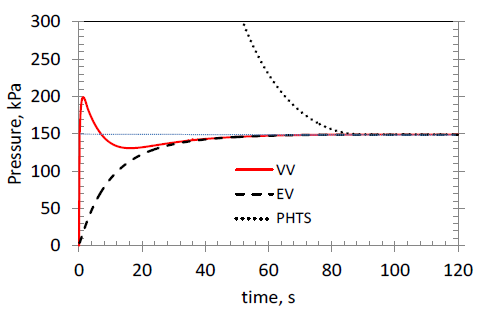Figure 4. EV “dry” solution pressure transient: total relief area 8 m2

## 5.png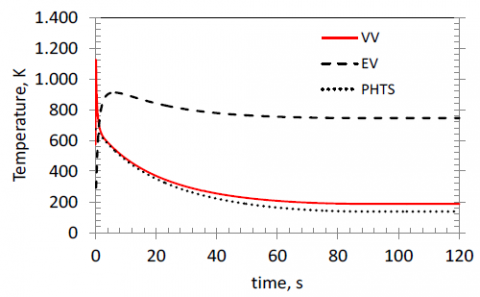Figure 5. EV “dry” solution temperature transient: total relief area 8 m2

## 6.pngFigure 6. EV “dry” solution flow rates: different relief area comparison

Figure 6 shows the flow rates between the volumes in the two cases with different relief area. Mass flow rate trends for “VV to EV” with larger discharge area (red) and “PHTS to VV” (dotted) are similar, and, being the two temperatures very similar, the initial pressure increment in the VV is interrupted at a lower value.

4. EV “DRY” Solution: Sensitivity Analyses

The parameters of the sensitivity calculations are summarized in Table 2.

Table 2.  Sensitivity analyses for the “dry” EV solution

 Case Break Area m2 EV volume m3 PHTS Temp K Heat source MW Total Relief Area m2 A1 0.2 120,000 673.15 0 8 A2 623.15 A3 723.15 B1 0.15 120,000 673.15 0 10 B2 0.25 6 A1_1 0.2 120,000 673.15 5 8 A1_2 10 A1_3 15 A1_1.1 0.2 134,000 673.15 5 8

In Figure 7 the results on the PHTS temperature sensitivity (A1, A2, A3) are shown. As the helium inventory and density are inversely proportional to the temperature, for an ideal gas, no sensible changes in the pressure transients are expected, as shown, as the flow rates and the total energy is the same in all the three cases.

Cases B1 and B2, where the break area is changed, show that if the relief area is changed proportionally, the pressure peak is the same as in the base case A1, with slight differences in the transient evolution over time (Figure 8).

The base case A1 has been evaluated considering no heat sources in the volume. A sensitivity on the heat source effects on the pressure transient has been performed, introducing 5, 10 or 15 MW (half in the VV and half in the PHTS). As the added energy introduced up to the pressure peak occurs is very low, with respect to the gas flow energy, the pressure peak is expected to be not influenced by the heat source. It affects the pressure increase over time, as shown in Figure 9. Calculations are interrupted when the temperature in a volume exceeds 1500 K, as for 10 MW and 15 MW.

To demonstrate the usefulness of Eq. (9), where a volume increment of the EV of 16,000 m3 for each MWh added, a further sensitivity has been done, case A1_1.1. A heat source of 5 MW has been introduced for 600 s (0.833 MWh) and the EV has been increased to 134,000 m3. As shown in Fig. 10, the pressure in the volumes after 600 s is 150 kPa, as expected.

## 7.pngFigure 7. EV “dry” solution pressure transient: PHTS temperature sensitivity

## 8.png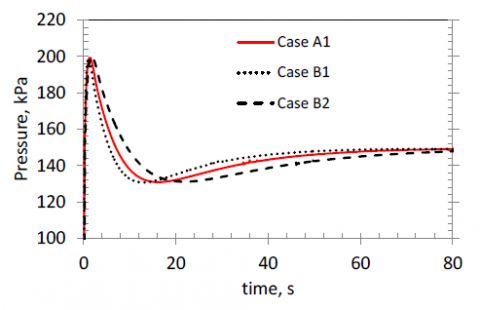Figure 8. EV “dry” solution pressure transient: break area sensitivity

## 9.png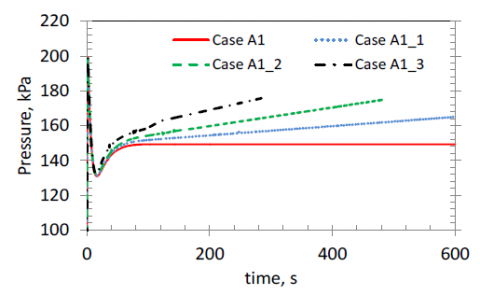Figure 9. EV “dry” solution pressure transient: heat source sensitivity

## 10.png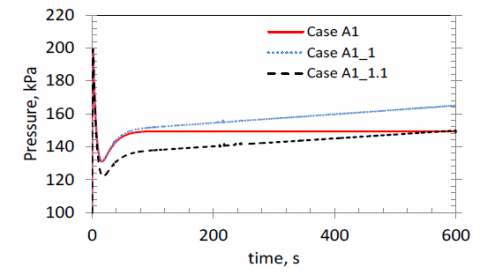Figure 10. EV “dry” solution pressure transient: heat source sensitivity

5. EV “WET” Solution: Sensitivity Analyses

In Figs. 11 and 12 are reported the results of pressure and temperatures transient calculation in case of “wet” Expansion Volume of 50,000 m3 plus 105 kg of cold water, (base case C1). The previously evaluated relief area of 8 m2 is used.

## 11.pngFigure 11. EV “wet” solution pressure transient: total relief area 8 m2

The pressure peak in the VV is 199.1 kPa at 1.25 s after the break. The equilibrium pressure (153 kPa, as expected from Eq. (10)) is reached at about 29 s.

The temperature transients in the VV and PHTS are like the “dry” solution. In the EV, the presence of saturated water limits the temperature, allowing a similar helium mass inventory as in the “Dry” case with a lower available volume.

Flow rates are shown in Fig. 13, and they are very similar to the “dry” solution with the same relief area.

The performed sensitivity analyses are summarized in Table 3, considering the “wet” solution for the EV.

## 12.pngFigure 12. EV “wet” solution temperature transient: total relief area 8 m2

## 13.pngFigure 13. EV “wet” solution, mass flow rates: total relief area 8 m2

Table 3. Sensitivity analyses for the “wet” EV solution

 Case Break Area m2 EV volume m3 EV Temp K Water mass kg PHTS Temp K Heat source MW Total Relief Area m2 C1 0.2 50,000 293.15 105 673.15 0 8 C2 54,000 623.15 C3 47,000 723.15 D1 0.15 50,000 293.15 105 673.15 0 10 D2 0.25 6 C1_1 0.2 50,000 293.15 105 673.15 5 8 C1_2 10 C1_3 15 C1_4 0.2 54,000 303.15 70,300 673.15 0 8 C1_5 59,700 313.15 13,900 C1_6 0.2 54,710 293.15 30,000 673.15 0 8 C1_7 46,455 3∙105

The EV free volume in cases C2 and C3 has been evaluated by Eq. (10), taking into account different initial temperature in the PHTS. Results in Figure 14 show the good performance of Eq. (10) in predicting the needed volume.

## 14.pngFigure 14. EV “wet” solution pressure transient: PHTS temperature sensitivity

## 15.pngFigure 15. EV “wet” solution pressure transient: break area sensitivity

As for the “dry” solution, a sensitivity on the area of the break has been performed (Figure 15, Cases D1 and D2), showing that with a relief area proportional to the break area the pressure peak can be limited to the required value.

Sensitivity analysis on the heat source is shown in Figure 16. In presence of water in the EV, pressure increasing over time is lower than in the case of a “dry” EV, but to limit the pressure the volume should be increased as described in the previous solution.

In Figure 17 results of the sensitivity analysis on the water temperature in the EV (C1_4 and C1_5) are shown. The EV size and the water inventory have been estimated as in Table 2, by Eqs. (10) and (11).

## 16.png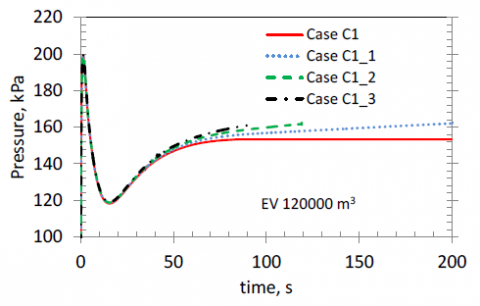Figure 16. EV “wet” solution pressure transient: heat source sensitivity

## 17.pngFigure 17. EV “wet” solution pressure transient: initial water temperature sensitivity

A water temperature increase at the equilibrium of 25 K has been assumed in these cases, as in the base case, therefore the size of the EV increases with the initial temperature of water, and the water inventory decreases (see Table 2). If a lower temperature increment is adopted, a higher water mass will be obtained from Eq. (11).

The results of CONSEN calculations show that Eqs. (10) and (11) are quite reliable and can be used for a preliminary design.

A last sensitivity has been performed about the water inventory in the EV. With the same EV size as in the base case C1 (50,000 m3), if the water inventory is different from the value obtained from Eq. (11), a different final pressure will be reached.

In Figure 18, with the same EV size as in the base case, the results adopting 3∙104 kg and 3∙105 kg of water are presented. As expected, lower is the mass in the EV, higher is the final pressure.

In order to obtain the required final pressure, the size of the EV has to be changed: decreased if the water inventory is high, and increased if the water inventory will be lower than the “optimum” value.

In Fig. 19 this is demonstrated: different sizes of the EV have been assumed (iterating between Eqs. (10) and (11), with different final temperatures in the EV) to reach the same final pressure with the two water inventories 3∙104 kg (EV free volume 54710 m3) and 3∙105 kg (EV free volume 46455 m3).

## 18.pngFigure 18. EV “wet” solution pressure transient: water inventory sensitivity at constant EV size

## 19.png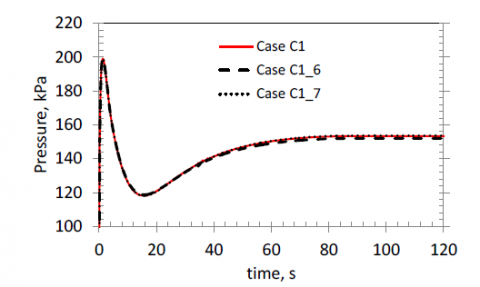Figure 19. EV “wet” solution pressure transient: water inventory sensitivity for different EV sizes

6. Conclusions

A methodology for a preliminary evaluation of the Expansion Volume and the relief pipes size, for a VVPSS to be adopted in a fusion reactor based on a Helium-cooled blanket, has been presented. The size of the EV depends on the total energy of the PHTS and it can be sized on the basis of a required final pressure at equilibrium, using simple energy balances. Two solutions have been analysed for the EV: a “dry” solution and a “wet” solution, where the use of cold water could greatly reduce the size of the volume and also better accommodate eventual heat sources.

In order to design a “dry” EV, Eq. (5) can be used, if no heat sources are present. If a heat source is considered, Eq. (8) could provide an estimated value of the volume for a given energy introduced. For the assumed conditions of the PHTS (2325 m3, pressure 8 MPa, temperature 673.15 K) and a volume of the VV = 2243 m3, without heat sources, a volume of 120,000 m3 for the EV is needed. If heat sources are present, an additional volume of 16,000 m3 per introduced MWh is a good estimation.

In “wet” EV solution, Eqs. (10) and (11) will provide the minimum free volume and the minimum amount of cold water to be used. In the above base case conditions, with water at 293.15 K, the minimum free volume and water inventory are 50,000 m3 and 105 kg, respectively. The Eqs. (10) and (11) provide values for different initial conditions.

Tables 2 and 3 summarize all the sensitivity analyses performed. In both cases, the total relief area between the VV and the EV is proportional to the break area and pressure of the PHTS. Eq (16) can estimate the minimum relief area to be adopted to limit the VV pressure at the required value (200 kPa). For a break area of 0.2 m2, a total relief area of 8 m2 has been evaluated in the PHTS conditions previously described.

The results obtained from a parametric study, using the computer code CONSEN, are in good agreement with the adopted methodology. Therefore, this can be useful to provide a first approximation of the system size, but the provided results have to be intended just as an indication in absence of reliable input data.

Acknowledgment

Authors thank Dr. Maria Teresa Porfiri – ENEA – for the fruitful discussion during the work and her invaluable comments on the manuscript.

Nomenclature
 A flow area, m2 cp specific heat at constant p, J.kg-1.K-1 cv specific heat at constant v, J.kg-1.K-1 E internal energy, J G mass velocity, kg.m-2.s-1 h specific enthalpy, J.kg-1 m mass, kg M molecular mass, kg.kmol-1 p pressure, Pa $Q ̇$ heat source, W t time, s T temperature, K u specific internal energy, J.kg-1 v specific volume, m3.kg-1 V volume, m3 x quality, - Greek symbols G mass flow rate, kg.s-1 g cp/ cv, - Subscripts BRK break crit critical EV Expansion Volume PHTS Primary Heat Transfer System RL relief VV Vacuum Vessel He helium l liquid sat saturation v steam w water 0 initial state f final state
References

 Bachmann et al. (2015). Initial DEMO tokamak design configuration studies, Fusion Eng Des, Vol. 98-99, pp. 1423-1426. DOI: 10.1016/j.fusengdes.2015.05.027

 Humrickhouse P.W., Merrill B.J. (2015). ARIES-ACT1 safety design and analysis, Fusion Sci Technol, Vol. 67, No. 1, pp. 167-178. DOI: 10.13182/FST14-799

 Caruso G., Giannetti F. (2016). Preliminary design of a pressure suppression system for in‐vessel components of a fusion reactor based on a water‐cooled blanket, Sci Technol Nucl Install, Article No. 8719695. DOI: 10.1155/2016/8719695

 Federici G., Kemp R., Ward D., Bachmann C., Franke T., Gonzalez S., Lowry C., Gadomska M., Harman J., Meszaros B., Morlock C., Romanelli F., Wenninger R. (2014). Overview of EU DEMO design and R&D activities, Fusion Eng Des, Vol. 89, No. 7-8, pp. 882-889. DOI: 10.1016/j.fusengdes.2014.01.070

 Liu H., Feng K. (2008). Thermal-hydraulic LOCA analysis of chinese helium-cooled solid breeder test blanket module for ITER, Fusion SciTechnol, Vol. 54, No. 4, pp. 970-977.

 Jin H.G., Lee D.W., Lee E.H., Kim S.K., Yoon J.S., Ahn M.Y., Cho S.Y. (2014). Scoping study on in-vessel LOCA of a Korean TBS in ITER, IEEE T Plasma Sci, Vol. 42, No. 3, Art. No. 6750105, pp. 671-674. DOI: 10.1109/TPS.2014.2303797

 Carloni D., Gonfiotti B., Paci S., Boccaccini L.V. (2015). LOCA accident for the demo helium cooled blanket, Fusion Sci Technol, Vol. 68, No. 2, pp. 353-357. DOI: 10.13182/FST14-924

 Gonfiotti B., Paci S. (2015). Normal and accidental scenarios analyses with MELCOR 1.8.2 and MELCOR 2.1 for the DEMO Helium-Cooled Pebble Bed blanket concept, Sci Technol Nucl Install, Art. No. 865829. DOI: 10.1155/2015/865829

 Sardain P., Ayrault L., Laffont G., Challet F., Marie L.B., Merrill B., Porfiri M.T., Caruso G. (2005). The EVITA programme: experimental and numerical simulation of a fluid ingress in the cryostat of a water-cooled fusion reactor, Fusion Eng Des, Vol. 75-79 (SUPPL.), pp. 1265-1269. DOI: 10.1016/j.fusengdes.2005.06.279

 Yamashita Y., Yokomine T., Ebara S., Shimizu A. (2004). Ice formation and pressurization under low pressure in cryostat, Fusion Sci Technol, Vol. 46, No. 4, pp. 541-546.

 Caruso G., Porfiri M.T. (2015). Ice layer growth on a cryogenic surface in a fusion reactor during a loss of water event, Prog Nucl Ener, Vol. 78, pp. 173-181. DOI: 10.1016/j.pnucene.2014.09.015

 Caruso G., Bartels H.W., Iseli M., Meyder R., Nordlinder S., Pasler V., Porfiri M.T. (2006). Simulation of cryogenic He spills as basis for planning of experimental campaign in the EVITA facility, Nucl Fusion, Vol. 46, No. 1, pp. 51-56. DOI: 10.1088/0029-5515/46/1/006

 Luo W., Li Y., Wang Q.H., Li J.L., Liao R.Q., Liu Z.L. (2016). Experimental study of gas-liquid two-phase flow for high velocity in inclined medium size tube and verification of pressure calculation methods, Int J Heat Technol, Vol. 34, No. 3, pp. 455-464. DOI: 10.18280/ijht.340315